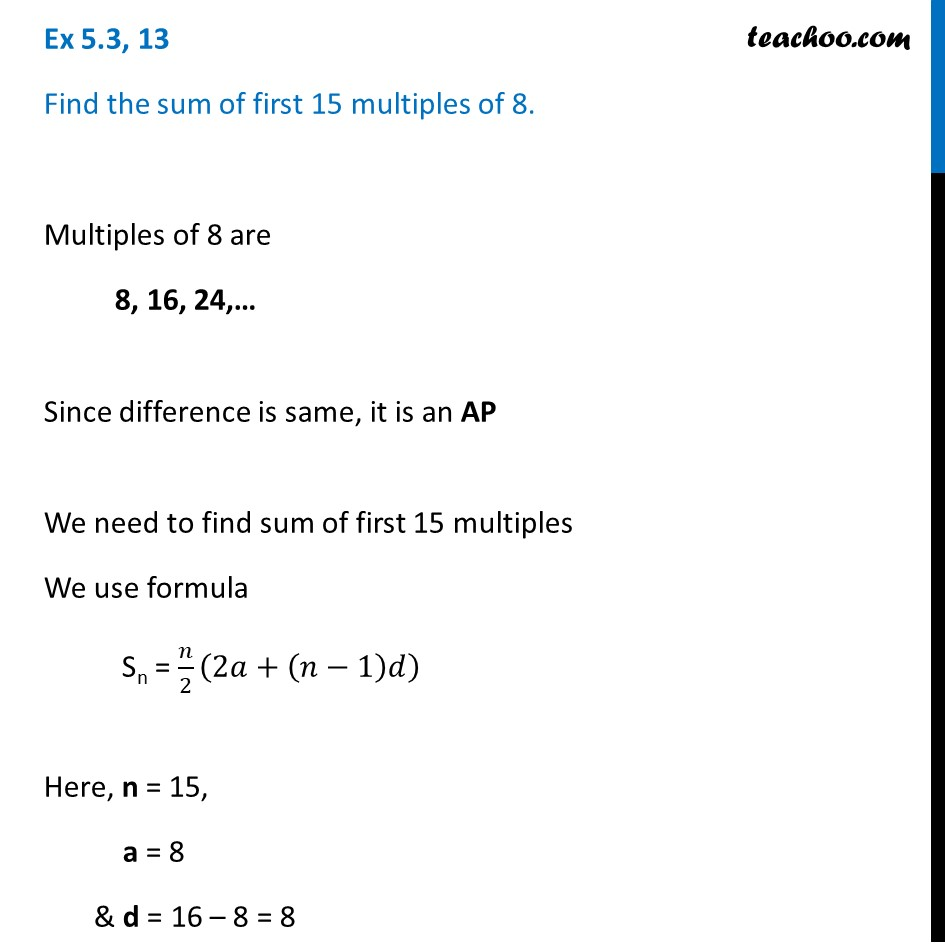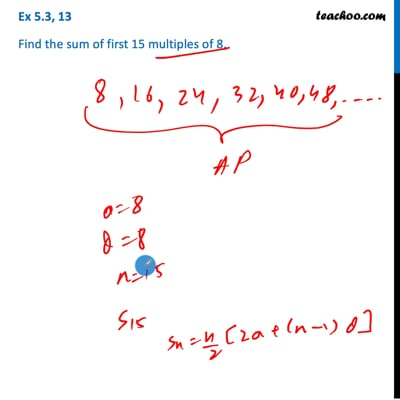Determining AP and finding sum

Chapter 5 Class 10 Arithmetic Progressions (Term 2)
Concept wiseThis video is only available for Teachoo black users

### Transcript

Ex 5.3, 13 Find the sum of first 15 multiples of 8. Multiples of 8 are 8, 16, 24,… Since difference is same, it is an AP We need to find sum of first 15 multiples We use formula Sn = 𝑛/2 (2𝑎+(𝑛−1)𝑑) Here, n = 15, a = 8 & d = 16 – 8 = 8 Putting values in formula Sn = 𝒏/𝟐 (𝟐𝒂+(𝒏−𝟏)𝒅) = 15/2(2 × 8+(15−1) × 8) = 15/2 (16+14 × 8) = 15/2 (16+112) = 15/2 × 128 = 960 Therefore, the sum of first 15 multiples of 8 is 960